How to Learn in 24 Hours?The Rapid Learning Movie

 Need Help? M-F: 9am-5pm(PST): Toll-Free: (877) RAPID-10 US Direct: (714) 692-2900 Int'l: 001-714-692-2900 24/7 Online Technical Support: The Rapid Support Center Secure Online Order:Need Proof? Testimonials by Our Users

 Rapid Learning Courses: MCAT in 24 Hours (2015-16) USMLE in 24 Hours (Boards) Chemistry in 24 Hours Biology in 24 Hours Physics in 24 Hours Mathematics in 24 Hours Psychology in 24 Hours SAT in 24 Hours ACT in 24 Hours AP in 24 Hours CLEP in 24 Hours DAT in 24 Hours (Dental) OAT in 24 Hours (Optometry) PCAT in 24 Hours (Pharmacy) Nursing Entrance Exams Certification in 24 Hours eBook - Survival Kits Audiobooks (MP3)

 Tell-A-Friend: Have friends taking science and math courses too? Tell them about our rapid learning system.Home »  Mathematics »  Pre-Calculus

Rational Functions

 Topic Review on "Title": Rational functions:  Any function which can be written of the form: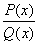where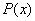and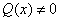are polynomials. Domain of a rational function: The set of points where the function is defined. Vertical asymptote: A line of the form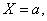such that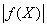takes arbitrarily large values near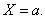Horizontal asymptote: A line of the form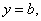such that if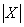tends to infinity,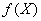tends to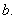The sum of rational functions: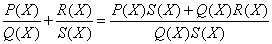. The product of rational functions: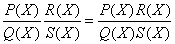. Simple functions Rational functions that cannot be reduced further. The division of rational functions: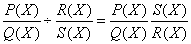.

Rapid Study Kit for "Title":
 Flash Movie Flash Game Flash Card Core Concept Tutorial Problem Solving Drill Review Cheat Sheet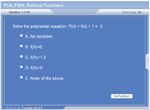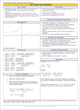"Title" Tutorial Summary : This tutorial describes the concept of rational functions.  These are a type of polynomial functions whose vertical and horizontal asymptotes helped describe the domain of rational functions. It is important to determine the degrees of the two functions that are part of a rational function so asymptotes could be determined.    This tutorial describes operations on rational functions through the use of examples. Simplification of rational functions is achieved by factoring the numerator function of rational functions and the denominator function of rational functions. Solving polynomial equations involves comparing the degrees and evaluating both functions so simplification can be achieved as much as possible.

 Tutorial Features: Specific Tutorial Features: • Simplification techniques are used to reduce rational functions. • Asymptotes are shown in the examples with the use of graphs. Series Features: • Concept map showing inter-connections of new concepts in this tutorial and those previously introduced. • Definition slides introduce terms as they are needed. • Visual representation of concepts • Animated examples—worked out step by step • A concise summary is given at the conclusion of the tutorial.

 "Title" Topic List: Basic definitions of rational functions Domain of rational functions Asymptotes of rational functions FormulaOperations on rational functions Simple rational functions Simplification of rational functionsPolynomial equations Solving polynomial equations

See all 24 lessons in Pre-Calculus, including concept tutorials, problem drills and cheat sheets:
Teach Yourself Pre-Calculus Visually in 24 Hours